Engineering Mechanics - Equilibrium of a Rigid Body

Why Engineering Mechanics Equilibrium of a Rigid Body?

In this section you can learn and practice Engineering Mechanics Questions based on "Equilibrium of a Rigid Body" and improve your skills in order to face the interview, competitive examination and various entrance test (CAT, GATE, GRE, MAT, Bank Exam, Railway Exam etc.) with full confidence.

Where can I get Engineering Mechanics Equilibrium of a Rigid Body questions and answers with explanation?

IndiaBIX provides you lots of fully solved Engineering Mechanics (Equilibrium of a Rigid Body) questions and answers with Explanation. Solved examples with detailed answer description, explanation are given and it would be easy to understand. All students, freshers can download Engineering Mechanics Equilibrium of a Rigid Body quiz questions with answers as PDF files and eBooks.

Where can I get Engineering Mechanics Equilibrium of a Rigid Body Interview Questions and Answers (objective type, multiple choice)?

Here you can find objective type Engineering Mechanics Equilibrium of a Rigid Body questions and answers for interview and entrance examination. Multiple choice and true or false type questions are also provided.

How to solve Engineering Mechanics Equilibrium of a Rigid Body problems?

You can easily solve all kind of Engineering Mechanics questions based on Equilibrium of a Rigid Body by practicing the objective type exercises given below, also get shortcut methods to solve Engineering Mechanics Equilibrium of a Rigid Body problems.

Exercise :: Equilibrium of a Rigid Body - General Questions

• Equilibrium of a Rigid Body - General Questions
1.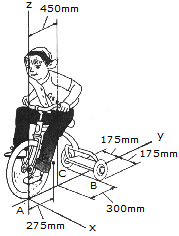The girl has a mass of 17kg and mass center at Gg, and the tricycle has a mass of 10kg and mass center at Gt. Determine the normal reactions at each wheel for equilibrium.

 A. NA = 14.77 N, NB = NC = 6.12 N B. NA = 128.8 N, NB = NC = 68.0 N C. NA = 144.9 N, NB = NC = 60.0 N D. NA = 13.15 N, NB = NC = 6.93 N

Explanation:

No answer description available for this question. Let us discuss.

2.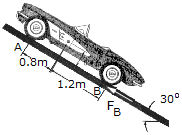The sports car has a mass of 1.5 Mg and mass center at G. If the front two springs each have a stiffness of kA=58 kN/m and the rear two springs each have a stiffness of kB = 65 kN/m, determine their compression when the car is parked on the 30° incline. Also, what frictional force FB must be applied to each of the rear wheels to hold the car in equilibrium?

 A. xA = 16.1 mm, xB = 42.2 mm, FB = 6.37 kN B. xA = 65.9 mm, xB = 39.2 mm, FB = 6.37 kN C. xA = 53.2 mm, xB = 50.5 mm, FB = 3.68 kN D. xA = 76.1 mm, xB = 45.3 mm, FB = 3.68 kN

Explanation:

No answer description available for this question. Let us discuss.

3.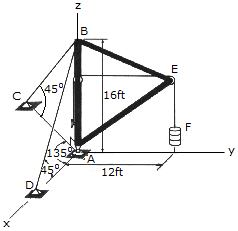Determine the tension in the supporting cables BC and BD and the components of reaction at the ball-and-socket joint A of the boom. The boom supports a drum having a weight of 200 lb. at F. Points C and D lie in the x—y plane.

 A. Ax = 0, Ay = 150 lb, Az = 562 lb, TBC = 300 lb, TBD = 212 lb B. Ax = 0, Ay = 150 lb, Az = 456 lb, TBC = 150 lb, TBD = 212 lb C. Ax = 0, Ay = 267 lb, Az = 843 lb, TBC = 533 lb, TBD = lb D. Ax = 0, Ay = 150 lb, Az = 500 lb, TBC = 212 lb, TBD = 212 lb

Explanation:

No answer description available for this question. Let us discuss.

4.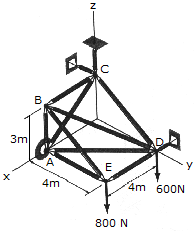The space truss is supported by a ball-and-socket joint at A and short links, two at C and one at D. Determine the x, y, z components of reaction at A and the force in each link.

 A. Ax = -1.050 kN, Ay = 1.050 kN, Az = 0.800 kN, Cy = -1.050 kN, Cz = 0.600 kN, Dx = 1.050 kN B. Ax = -1.400 kN, Ay = 1.400 kN, Az = 0.800 kN, Cy = -1.400 kN, Cz = 0.600 kN, Dx = 1.400 kN C. Ax = -2.49 kN, Ay = 1.867 kN, Az = 0.800 kN, Cy = -2.49 kN, Cz = 0.600 kN, Dx = 2.49 kN D. Ax = -1.867 kN, Ay = 1.867 kN, Az = 0.800 kN, Cy = -1.867 kN, Cz = 0.600 kN, Dx = 1.867 kN

Explanation:

No answer description available for this question. Let us discuss.

5.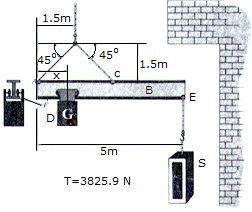The flying boom B is used with a crane to position construction materials in coves and underhangs. The horizontal "balance" of the boom is controlled by a 250-kg block D, which has a center of gravity at G and moves by internal sensing devices along the bottom flange F of the beam. Determine the position x of the block when the boom is used to lift the stone S, which has a mass of 60 kg. The boom is uniform and has a mass of 80 kg.

 A. x = 2.500 m B. x = 0.340 m C. x = 1.180 m D. x = 0.600 m